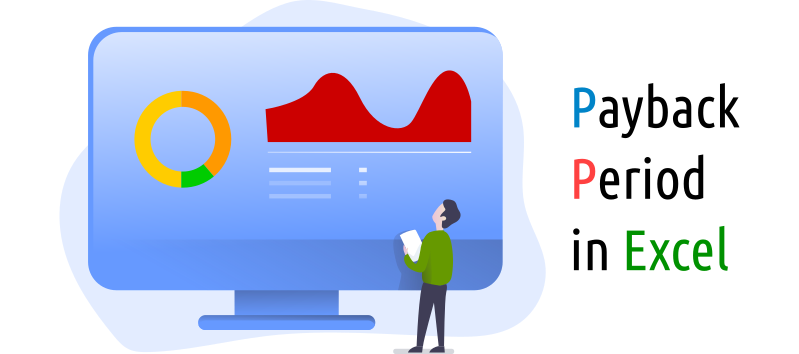# How to Calculate the Payback Period in Excel

The payback period is the time it takes for a project to recover the investment cost. For example, if you invest \$100 and the returns are \$50 per year, you will recover your initial investment in two years.# NPV Formula in Excel

The NPV formula in Excel is counterintuitive at first. When I first used it, I made a simple mistake by selecting all the cash flow, including the initial investment. I learned that Excel requires you to select only the future flows and then discount the initial investment from the result, to get the accurate NPV value.

# IRR calculation in Excel

This article teaches you how to calculate the IRR (Internal Return Rate) using Excel. The Excel function to calculate the IRR is “IRR” and it’s really simple, since it only takes the range that contains the flow of funds, including the initial investment.